### Puzzling Greys

This page has now been moved to Experiments with Colour and Brightnesses.
A puzzle because formulas for converting to greyscale do not seem to give a consistent level of brightness. This page demonstrates that and then, at the foot of the page, explains why that is.
From the documentation for Gimp, we find three formulas for converting a coloured image to greyscale:
Lightness
The graylevel will be calculated as
Lightness = ½ × (max(R,G,B) + min(R,G,B))
Luminosity
The graylevel will be calculated as
Luminosity = 0.21 × R + 0.72 × G + 0.07 × B
Average
The graylevel will be calculated as
Average Brightness = (R + G + B) ÷ 3
The formula that Gimp calls luminosity is close to the formula for rendering an image in the CIE 1921 XYZ colorspace, so we’ll refer to that as the XYZ formula.
Let’s look at each of the conversion formulas applied to text over a black background:
First, the Lightness formula. Each of these text colours gives a lightness value of 137:
 RGB values are 224,50,202 RGB values are 86,121,188 RGB values are 255,177,19 RGB values are 173,111,101
And Luminosity (XYZ) formula. Each of these gives a luminosity value of 137:
 RGB values are 253,102,139 RGB values are 38,168,125 RGB values are 238,117,28 RGB values are 122,143,125
And the Average formula. Each of these four examples gives a R+G+B total of 291:
 RGB values are 13,236,42 RGB values are 131,88,72 RGB values are 243,17,31 RGB values are 35,92,164
The four text colours in each row are clearly not all the same level of brightness, or greyscale, which equates to brightness.
So while each formula will convert to a satisfactory-looking greyscale image on a photograph, that is because the levels of grey among the pixels look sufficiently distinct from each other, relatively; none of those formulas is especially good at providing a precise greyscale level for a given RGB colour triad.
I should mention that I am not the only one to have pointed this out, see Rendering in XYZ color space and why this is wrong.
Here’s what Photoshop (version 5.5) makes of these text colour values as blocks converted to grayscale.
First, lightness, at #E032CA, #5679BC, #FFB113, #AD6F65: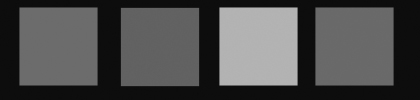Luminosity (CIE XYZ) at #FD668B, :#26A87D, #EE751C, #7A8F7D: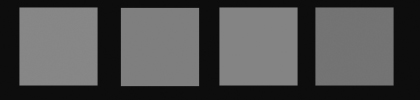Average at #0DEC2A, #835848, #F3111F, #235CA4: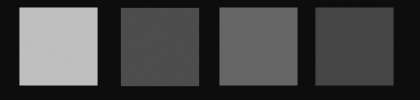This may look like Photoshop is using the luminosity calculation to convert to greyscale, but it isn’t; if we put those four luminosity blocks together (clockwise from the left) we can see that it is not:Maybe it’s using a YIQ brightness calculation as is the recommended method in the W3C Web Usability Guidelines. Here are four blocks calculated with the YIQ formula of R × 0.299 + G × 0.587 + B × 0.114, each giving a YIQ total of 97:
 RGB values are 247,23,79 RGB values are 107,94,88 RGB values are 211,19,202 RGB values are 20,121,175
Is Photoshop using that? Nope:But Photoshop looks like it is getting the greyscale conversion about right. Here is a block produced in Photoshop showing red, green and blue at values 255, 192, 128 and 64: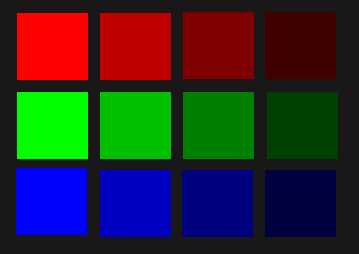And now I am going to convert that to greyscale and see what grey values Photoshop gives us.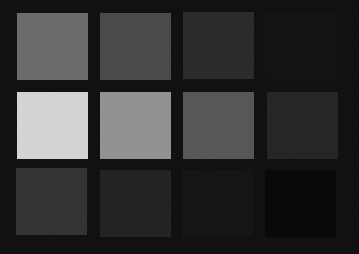That looks pretty good, perceptually, it goes like this:
 Colour value Red % Green % Blue % 255 130 50.9804% 220 86.2745% 70 27.4510% 192 96 50.0000% 165 85.9375% 50 26.0417% 128 62 48.4375% 110 85.9375% 30 23.4375% 64 28 43.7500% 54 84.3750% 10 15.6520%
On my page Formulas for Calculating Colour Brightness, I show a formula that emulates as close as makes no difference what Photoshop is doing. I also point out on that page that for the purposes of determining relative brightness – in other words a consistent greyscale – you need to weight the formulas to account for the phenomenon of lighter colours being easier to distinguish at a given brightness value difference than darker ones.
There are a number of pages that demonstrate the effect of this:
Colour Brightness Experiment shows the results of various methods of calculating brightness, with and without a brightness weighting.
Text Readability in Colour demonstrates the effect of text over a background in selectable colours from the full RGB range of the monitor, showing their brightness levels according to various calculation criteria.
Eighteen Shades of Greyscale shows how variable greyscale conversion can be using different calculation criteria.
Greyscale Conversions shows by colour-pick, side-by-side, the difference in greyscale conversion for a selected hue.
So there we are, not such a puzzle after all, the confusion turns out to be caused by those who insist upon promoting a linear calculation for something that is not linear.